Test: Basic Concepts - 2

# Test: Basic Concepts - 2

Test Description

## 10 Questions MCQ Test Network Theory (Electric Circuits) | Test: Basic Concepts - 2

Test: Basic Concepts - 2 for Electrical Engineering (EE) 2022 is part of Network Theory (Electric Circuits) preparation. The Test: Basic Concepts - 2 questions and answers have been prepared according to the Electrical Engineering (EE) exam syllabus.The Test: Basic Concepts - 2 MCQs are made for Electrical Engineering (EE) 2022 Exam. Find important definitions, questions, notes, meanings, examples, exercises, MCQs and online tests for Test: Basic Concepts - 2 below.
Solutions of Test: Basic Concepts - 2 questions in English are available as part of our Network Theory (Electric Circuits) for Electrical Engineering (EE) & Test: Basic Concepts - 2 solutions in Hindi for Network Theory (Electric Circuits) course. Download more important topics, notes, lectures and mock test series for Electrical Engineering (EE) Exam by signing up for free. Attempt Test: Basic Concepts - 2 | 10 questions in 30 minutes | Mock test for Electrical Engineering (EE) preparation | Free important questions MCQ to study Network Theory (Electric Circuits) for Electrical Engineering (EE) Exam | Download free PDF with solutions
 1 Crore+ students have signed up on EduRev. Have you?
Test: Basic Concepts - 2 - Question 1

### Twelve 6Ω resistor are used as edge to form a cube. The resistance between two diagonally opposite corner of the cube is

Detailed Solution for Test: Basic Concepts - 2 - Question 1

The current i will be distributed in the cube branches symmetrically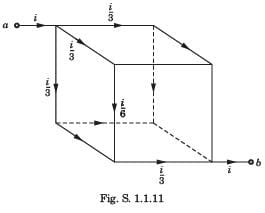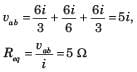Test: Basic Concepts - 2 - Question 2

### v1 = ?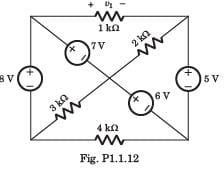Detailed Solution for Test: Basic Concepts - 2 - Question 2

If we go from +side of 1 kΩ through 7 V, 6 V and 5V,weget v1 = 7+6 + 5 = 8 V

Test: Basic Concepts - 2 - Question 3

### The voltage vo in fig. P1.1.11 is always equal to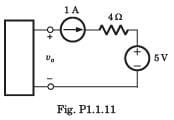Detailed Solution for Test: Basic Concepts - 2 - Question 3

It is not possible to determine the voltage across 1 A source.

Test: Basic Concepts - 2 - Question 4

Req = ?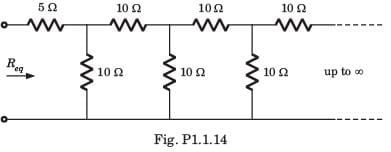Detailed Solution for Test: Basic Concepts - 2 - Question 4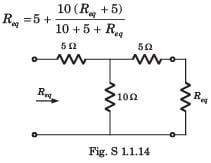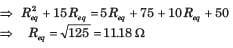Test: Basic Concepts - 2 - Question 5

In the circuit of fig. P.1.1.31 dependent source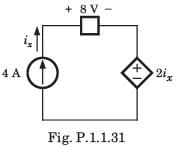Detailed Solution for Test: Basic Concepts - 2 - Question 5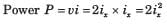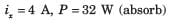Test: Basic Concepts - 2 - Question 6

A capacitor is charged by a constant current of 2 mA and results in a voltage increase of 12 V in a 10 sec interval. The value of capacitance is

Detailed Solution for Test: Basic Concepts - 2 - Question 6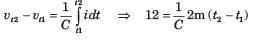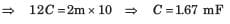Test: Basic Concepts - 2 - Question 7

The energy required to charge a 10 μF capacitor to 100 V is

Detailed Solution for Test: Basic Concepts - 2 - Question 7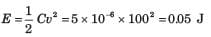Test: Basic Concepts - 2 - Question 8

The current in a 100 μF capacitor is shown in fig. P.1.1.34. If capacitor is initially uncharged, then the waveform for the voltage across it is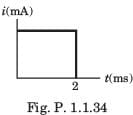Detailed Solution for Test: Basic Concepts - 2 - Question 8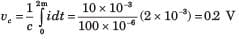This 0.2 V increases linearly from 0 to 0.2 V. Then current is zero. So capacitor hold this voltage.

Test: Basic Concepts - 2 - Question 9

The voltage across a 100 μF capacitor is shown in fig. P.1.1.35. The waveform for the current in the capacitor is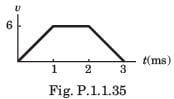Detailed Solution for Test: Basic Concepts - 2 - Question 9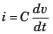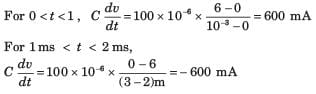Test: Basic Concepts - 2 - Question 10

The waveform for the current in a 200 μF capacitor is shown in fig. P.1.1.36 The waveform for the capacitor voltage is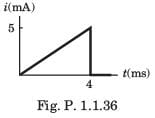Detailed Solution for Test: Basic Concepts - 2 - Question 10

For 0 ≤ t ≤ 4,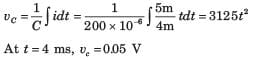It will be parabolic path. at t = 0 t-axis will be tangent.

## Network Theory (Electric Circuits)

23 videos|21 docs|27 tests
 Use Code STAYHOME200 and get INR 200 additional OFF Use Coupon Code
Information about Test: Basic Concepts - 2 Page
In this test you can find the Exam questions for Test: Basic Concepts - 2 solved & explained in the simplest way possible. Besides giving Questions and answers for Test: Basic Concepts - 2, EduRev gives you an ample number of Online tests for practice

## Network Theory (Electric Circuits)

23 videos|21 docs|27 tests